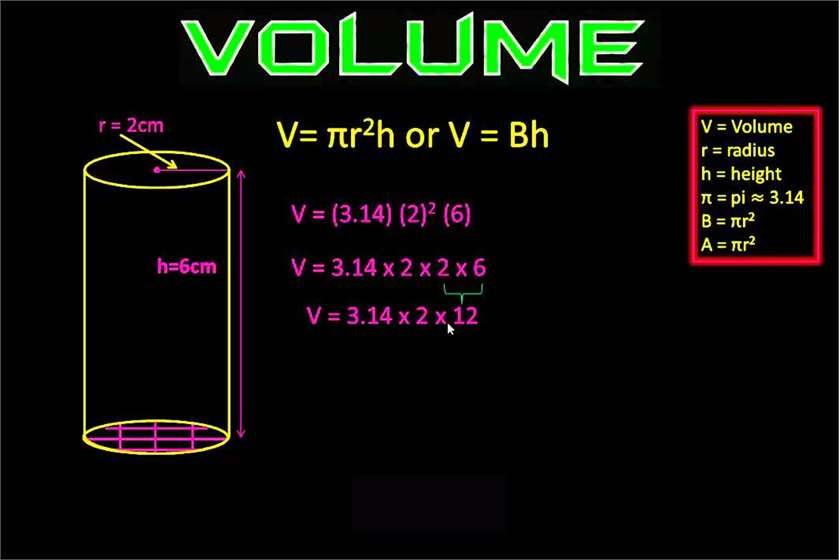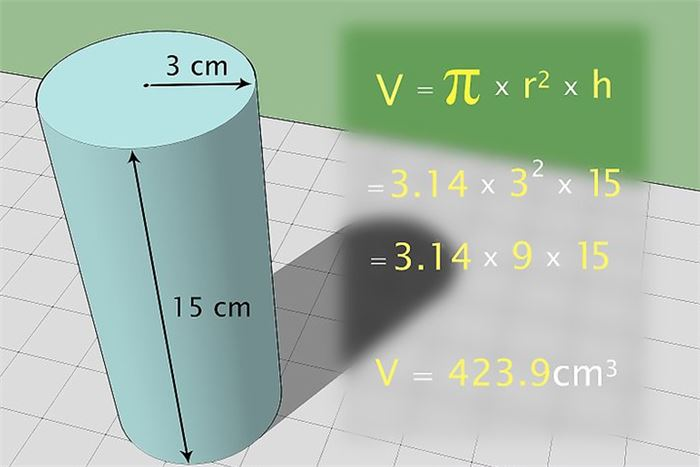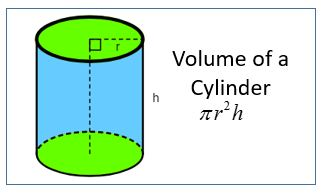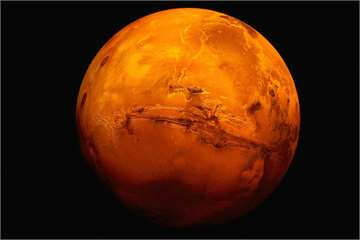# How to Find the Volume of a Cylinder?

1,765 Views Updated: 23 Aug 2017While many would say that finding the area of a circle, rectangle, square and other 2-dimensional shapes is a piece of cake, the problem arises when you are dealing with 3-dimensional shapes.

A cylinder is a closed solid formed when two parallel, congruent circles are connected using a curved surface. How do you find the volume of a cylinder? Well, if you would like to know how then here are simple steps you need to follow.

#1. Find The Radius Of The Circular Base

Since the two circles at the two flat edges of a cylinder have the same radius, you only need to know the radii of only one of these. In case it is unknown, then the first option is to measure it using a ruler. If you know the diameter of the circle, then you can find the radius from it by dividing it by 2, since the radius is always half the diameter. The radius can also be derived if the circumference of the circle is known by using the formula, circumference = 2πr.(Image Courtesy: Math Lab)

#2. Find The Area Of The Circle

Once you have successfully figured out the radius of the circle at the base, the next step is to calculate the area of the circle. You can conveniently measure it using the formula πr*r, where ‘r’ is the radius of the circle, and ‘π’ is a mathematical constant whose value is 3.14159 or 22/7. You do not necessarily have to calculate the exact value of the constant, and you can also leave the answer in terms of ‘π.'

#3. Find The Height Of The Cylinder

After finding the area of the circle at the base, now you need to know the height of the cylinder. The height of the cylinder is the distance between the edges of the two bases or the circles at the corner of the cylinder. In case you do not know it, then one way to measure it is using a ruler. Furthermore, if you know the curved surface area of the cylinder, then you can ascertain the cylinder’s height from it using the formula, curved surface area = 2πrh.

#4. Multiply The Area Of The Circle With The Height Of The Cylinder

Once you have calculated the area of the circle at the base as well as the height of the cylinder, what you need to do next is multiply the two, and you will get its volume. Think of the volume of the cylinder as the volume of the circle at the base stretching throughout the length or height of the cylinder. But since a circle is a 2-dimensional shape, its area is its volume. Therefore, multiplying the two with each other, you get the volume of the cylinder.(Image Courtesy: Online Math Learning)

It is also important to note that volume is always calculated in cubic units, such as cubic cm, cubic inches, cubic feet and cubic meter. Also, always make sure that all your measurements are in the same unit before you start calculating the volume. So, if the radius and height are in other units, convert them into the same and then start your calculation.

Did the article help you understand the concept better or was there something else you were looking for? We love to hear from you so share your thoughts and opinion with us using the comment section below.Posted by: Julia Posts: (3) Opinions: (8) Points: 350 Rank: 499

#### Related polls### Is 'we use 10 Percent of our Brain' a Myth?

23 Opinions### If Mars has Water, Does that mean it can support Life Forms as well?

27 Opinions8 Opinions